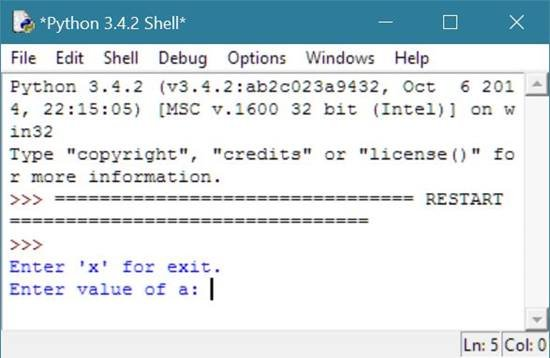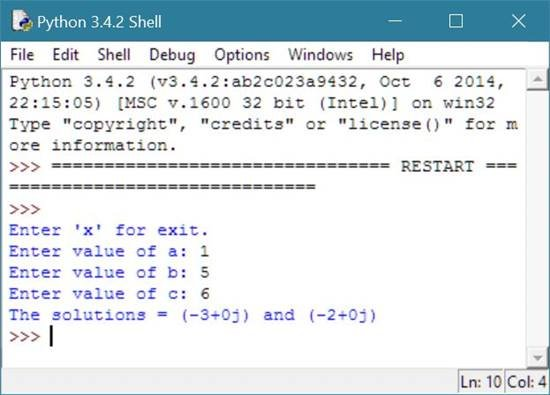# Python Program to Solve Quadratic Equation

## Solve Quadratic Equation in Python

To solve quadratic equation in python, you have to ask from user to enter the value of a, b, and c. Now calculate the value of d, and finally calculate the value of r1 and r2 to solve the quadratic equation of the given value of a, b, and c as shown in the program given below.

## Python Programming Code to Solve Quadratic Equation

Following python program ask from the user to enter the value of a, b, and c to solve the quadratic equation and print the result as output:

```# Python Program - Solve Quadratic Equation

import cmath;
print("Enter 'x' for exit.");
num1 = input("Enter value of a: ");
if num1 == 'x':
exit();
else:
num2 = input("Enter value of b: ");
num3 = input("Enter value of c: ");
number1 = float(num1);
number2 = float(num2);
number3 = float(num3);
d = (number2**2) - (4*number1*number3);
r1 = (-number2-cmath.sqrt(d))/(2*number1);
r2 = (-number2+cmath.sqrt(d))/(2*number1);
print("The solutions = {0} and {1}" .format(r1,r2));
```

Here is the sample run of the above python program shows how to solve quadratic equation:

This is the initial output, shows you to enter either for exit (that is x) or for the values of all the three variables (that is a, b, and c):Now we are going to enter the value of a as 1, b as 5 and c as 6 to solve quadratic equation with these values:Here is the same program written and run on python shell:Tools
Calculator